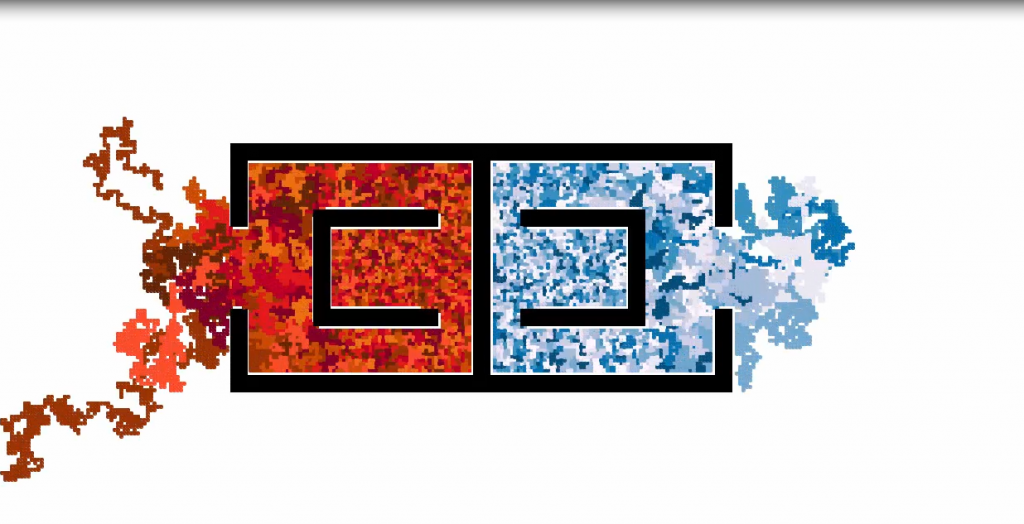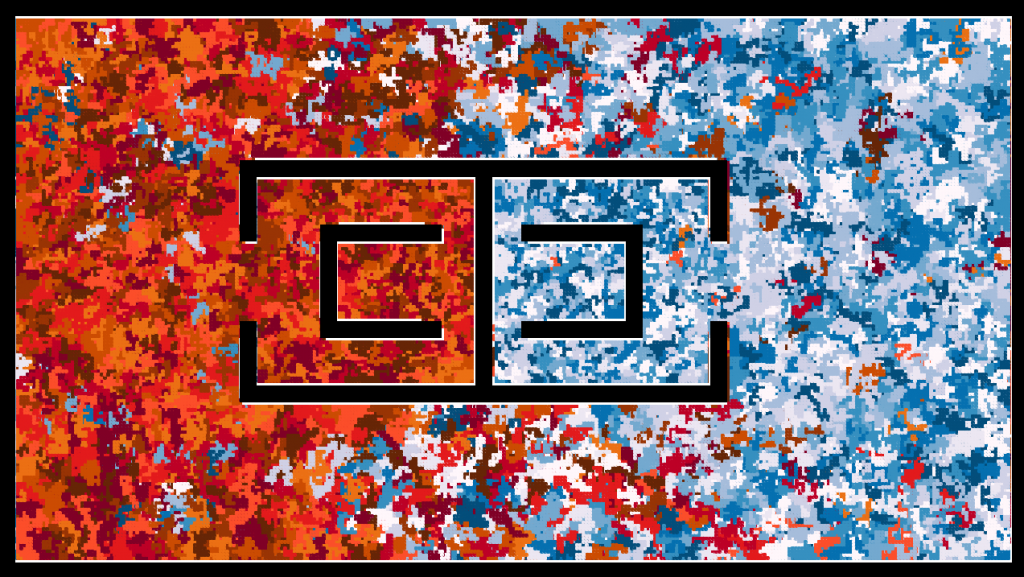# icy hot gaussian dance

Silly project using processing 3.0. It’s essentially the same as my previous one(snakes maze) but with more colors.

Rules

• snake/snake interactions overlap, nothing happens.
• snake/wall interaction = if a snake is headed in the direction of a wall, it turns around and heads in the opposite direction.Full screen it! (WARNING: I’ve received a multitude of negative reviews about the music chosen. Also, note that youtube and vimeo compression does not look like the original, especially when smaller snake widths are chosen.) The video is sped up ~400%.

update
Someone asked me how long it would take 1 snake to travel to the other snake color group’s starting point. So I ran the experiment and responded with:

It took my computer approximately ~27 minutes for one snake to reach the other color group’s starting point. Here’s a picture:You can see a snake from the red group infiltrated blue’s base. Blue group almost won, got really close, but turned around last minute and lost interest in the objective.

I was thinking, perhaps this could be turned into some sort of high stakes casino game.

Patrons would place bets on either side, and the casino would take a rake from the total pot. Each “red vs. blue” event would be displayed on a huge monitor in a palatial area. The color groups could also be adjusted to match the theme/scheme palette of the casino.

It would be interesting to see a group of people, gathered around, financially invested, yelling angerly at some randomized color generation.

All I need is 1 giant flat screen TV, some sort of cheap dell server, a gambling licence, a marble stone coliseum structure, and electricity,to make my vision a reality.

```public int snakes = 2000; public int[] numholdx = new int[snakes]; public int[] numholdy = new int[snakes]; public color[] colorhold = new color[snakes]; void setup() { int q = 0; for (int k = 0 ; k < snakes; k++) { q+=1; if (k > 999) { numholdx[k] = 490; numholdy[k] = 360; } else { numholdx[k] = 705; numholdy[k] = 360; } } int swap = 0; for (int k = 0 ; k < snakes; k++) { if (k > 999) { switch(swap) { case 0: colorhold[k] = #800026; break; case 1: colorhold[k] = #bd0026; break; case 2: colorhold[k] = #e31a1c; break; case 3: colorhold[k] = #fc4e2a; break; case 4: colorhold[k] = #ec7014; break; case 5: colorhold[k] = #cc4c02; break; case 6: colorhold[k] = #993404; break; case 7: colorhold[k] = #662506; break; } swap +=1; if ( swap == 8 ) swap = 0; } else { switch(swap) { case 0: colorhold[k] = #fff7fb; break; case 1: colorhold[k] = #ece7f2; break; case 2: colorhold[k] = #d0d1e6; break; case 3: colorhold[k] = #a6bddb; break; case 4: colorhold[k] = #74a9cf; break; case 5: colorhold[k] = #3690c0; break; case 6: colorhold[k] = #0570b0; break; case 7: colorhold[k] = #034e7b; break; } swap +=1; if ( swap == 8 ) swap = 0; } } size(1280, 720); background(#ffffff); }   void draw() { stroke(000); fill(000); //maze walls rect(0, 0, 20, 720); rect(1260, 0, 20, 720); rect(0, 0, 1280, 20); rect(0, 700, 1280, 20); rect(300, 200, 600, 20); rect(300, 480, 600, 20); rect(300, 200, 20, 100); rect(885, 200, 20, 100); rect(300, 400, 20, 100); rect(885, 400, 20, 100); rect(593, 200, 20, 300); rect(400, 280, 150, 20); rect(400, 400, 150, 20); rect(400, 280, 150, 20); rect(400, 400, 150, 20); rect(400, 300, 20, 100); rect(780, 300, 20, 100); rect(650, 280, 150, 20); rect(650, 400, 150, 20); rect(400, 400, 150, 20);   for ( int line = 0; line < snakes; line ++) { fill(colorhold[line]); stroke(colorhold[line]); int r = int(random(0,4)); //random direction. int len_max = int(random(0,5)); //direction. int keep_dir = r; //box size. int size = 4; if ( keep_dir == 1 && get(numholdx[line], numholdy[line]- size) == -16777216) keep_dir = 3; else if ( keep_dir == 3 && get(numholdx[line], numholdy[line] + size + size) == -16777216) keep_dir = 1; else if ( keep_dir == 0 && get(numholdx[line] + size + size, numholdy[line]) == -16777216) keep_dir = 2; else if ( keep_dir == 2 && get(numholdx[line] - size, numholdy[line]) == -16777216) keep_dir = 0; if ( keep_dir == 0) { rect( numholdx[line] + size, numholdy[line], size, size, 2); numholdx[line] += size; } else if ( keep_dir == 3) { rect( numholdx[line], numholdy[line] + size,size, size, 2); numholdy[line] += size; } else if ( keep_dir == 2) { rect( numholdx[line] - size, numholdy[line], size, size, 2); numholdx[line] -= size; } else if ( keep_dir == 1) { rect( numholdx[line], numholdy[line]- size,size, size, 2); numholdy[line] -= size; } } }```